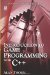# 2.1 What Is Algebra?

## 2.1 What Is Algebra?

Algebra expands upon the ideas considered in arithmetic, such as those covered in the previous chapter. There we added, multiplied, subtracted, and divided numbers that we could both count and measure. These were known quantities; numbers such as 1, 2, 15, 9, etc. In algebra, we deal with known quantities just like the previous chapter, but we also deal with unknown numbers. These unknowns are usually what we aim to find. The unknown numbers are replaced by letters such as x, y, or z. For example: a + b = c is an example of algebra at work. Some number a, when added to b, will equal c. We do not know the numbers, but we know that, were we to replace them with some numbers of our choosing there would be an infinite number of possible combinations. For example, a could be 5 and b could be 3, in which case, since a + b = c, c is 8. As this chapter progresses I hope the importance of representing numbers using letters will become apparent through real examples. One of the first topics to consider in algebra is the fundamental rules for working with algebraic expressions (like a + b = c).Introduction to Game Programming with C++ (Wordware Game Developers Library)
ISBN: 1598220322
EAN: 2147483647
Year: 2007
Pages: 225
Authors: Alan Thorn

Similar book on Amazon

flylib.com © 2008-2017.
If you may any questions please contact us: flylib@qtcs.net